# Data sampling

TableTorch’s data sampling function reads the data of the selected range and inserts a new sheet containing separate samples of original data with rows being selected in accordance with specified options.

It can be used for the following purposes:

• Splitting the data into separate train-test sets or a number of equally sized sets useful for K-Fold Cross-Validation.
• Randomizing rows order.
• Stratified random sampling:
• Uniform: the splits should have the same number of rows belonging to each stratum.
• Proportional: the share of each stratum should be the same as it was in the original dataset in every sample.
• Sampling with replacement: each row has same probability of being included in resulting split, number of rows can be greater than it is in original dataset, there is a chance that the same row appears more than once in particular sample.

The sampling techniques that are present on the Sampling panel are the same as they are for linear and logistic regressions. The underlying algorithm is also the same. Thus, sampling could be useful to visually review how the data is going to be split before doing a regression, as well as to perform any other research on the samples.

Let’s review application of each of the available options on the vehicle dataset in the following sections.

## Start TableTorch

1. Install TableTorch to Google Sheets via Google Workspace Marketplace. More details on initial setup.
2. Click on the TableTorch iconon right-side panel of Google Sheets.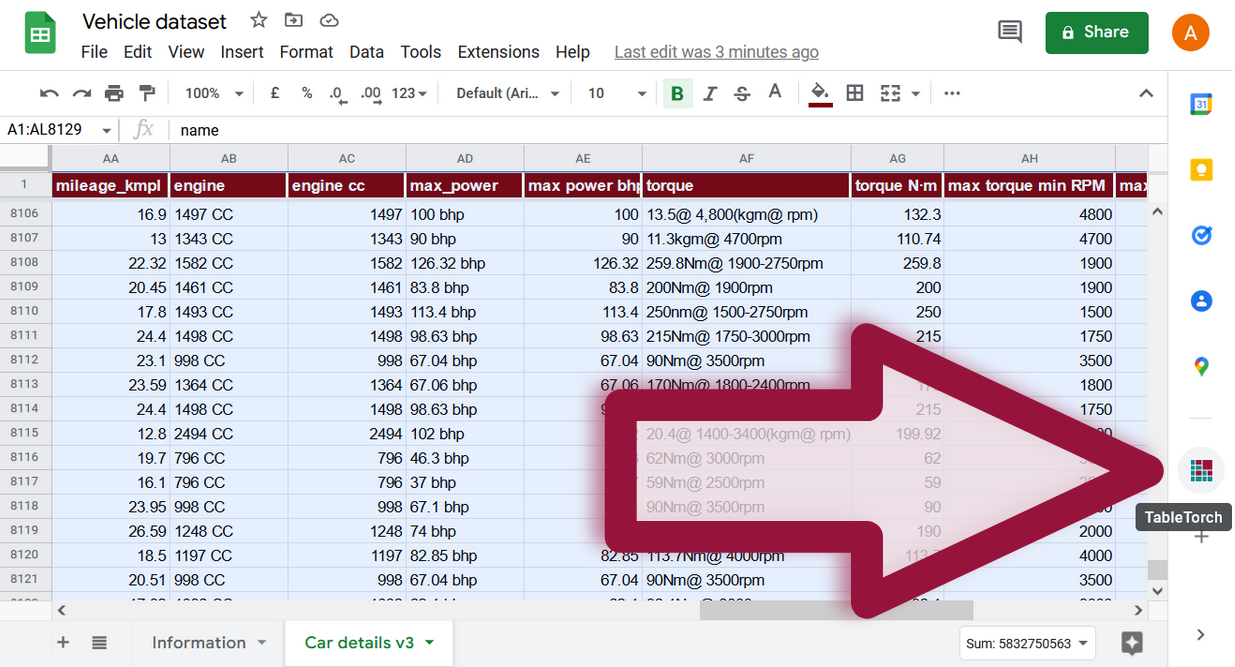## Train-test split

Select the whole dataset and click on the Sampling button of the TableTorch menu.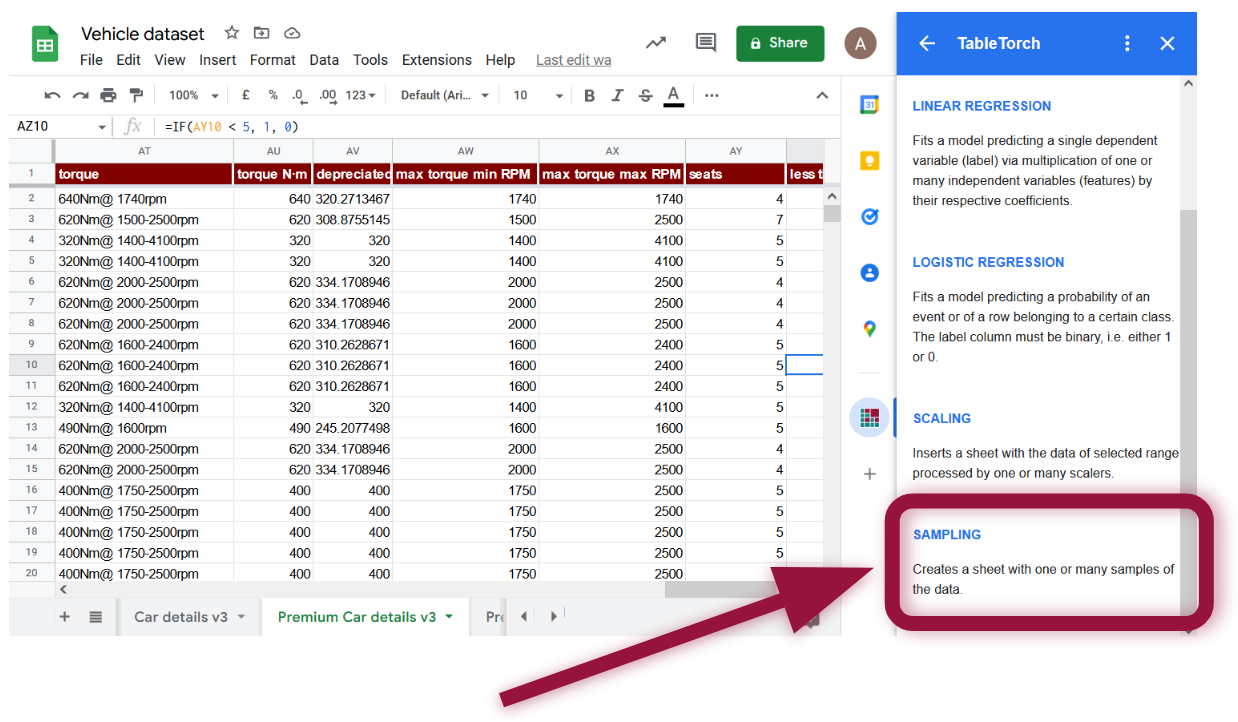A panel with sampling options will appear: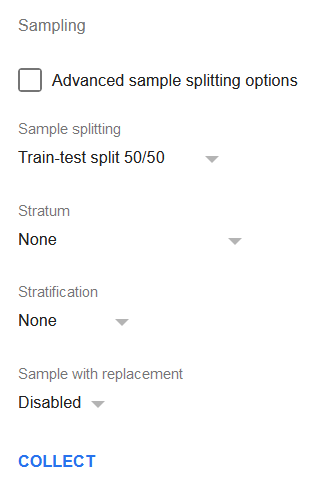Click the Collect button and TableTorch will insert a new sheet with two samples of the data, each consisting of half of the rows of the original dataset.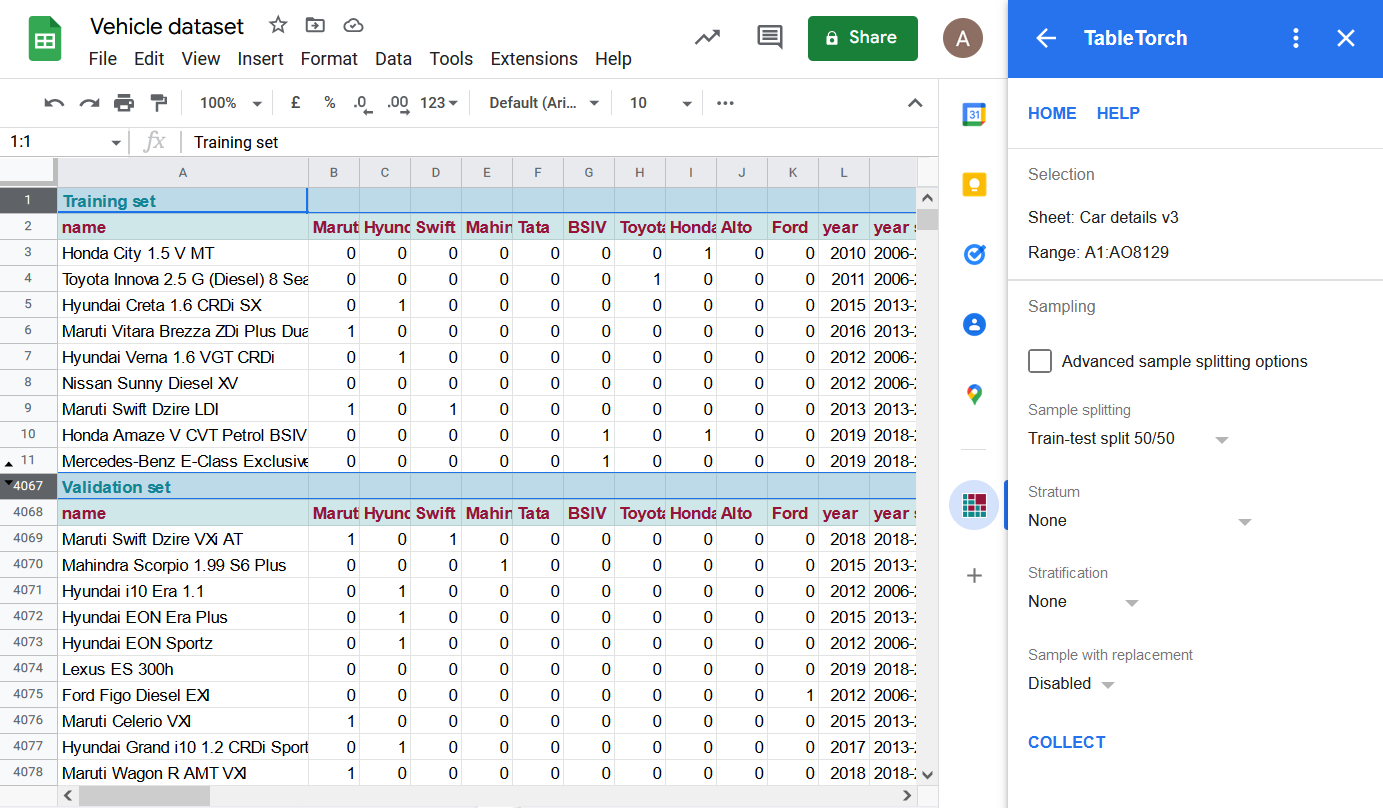Rows 12..4066 are hidden on the screenshot above in order to demonstrate that the resulting sheet contains two separate sets of data with identical column structure, a header row with set’s identification, as well as an additional row with names of the columns.

Sampling with replacement was not used so each of the sets contains only unique records from original dataset.

## Stratified 3-Fold Cross-Validation splitting

Let’s try 3-Fold Cross Validation splitting with year stratum (see fine-tuning regressions page for its formula) as the stratum column and stratified uniform random sampling. The entirety of options set is shown on the picture below.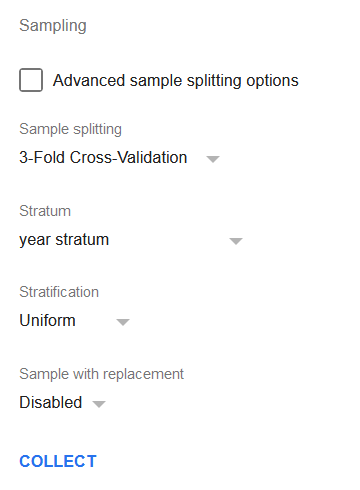Click the Collect button in order to perform the sampling.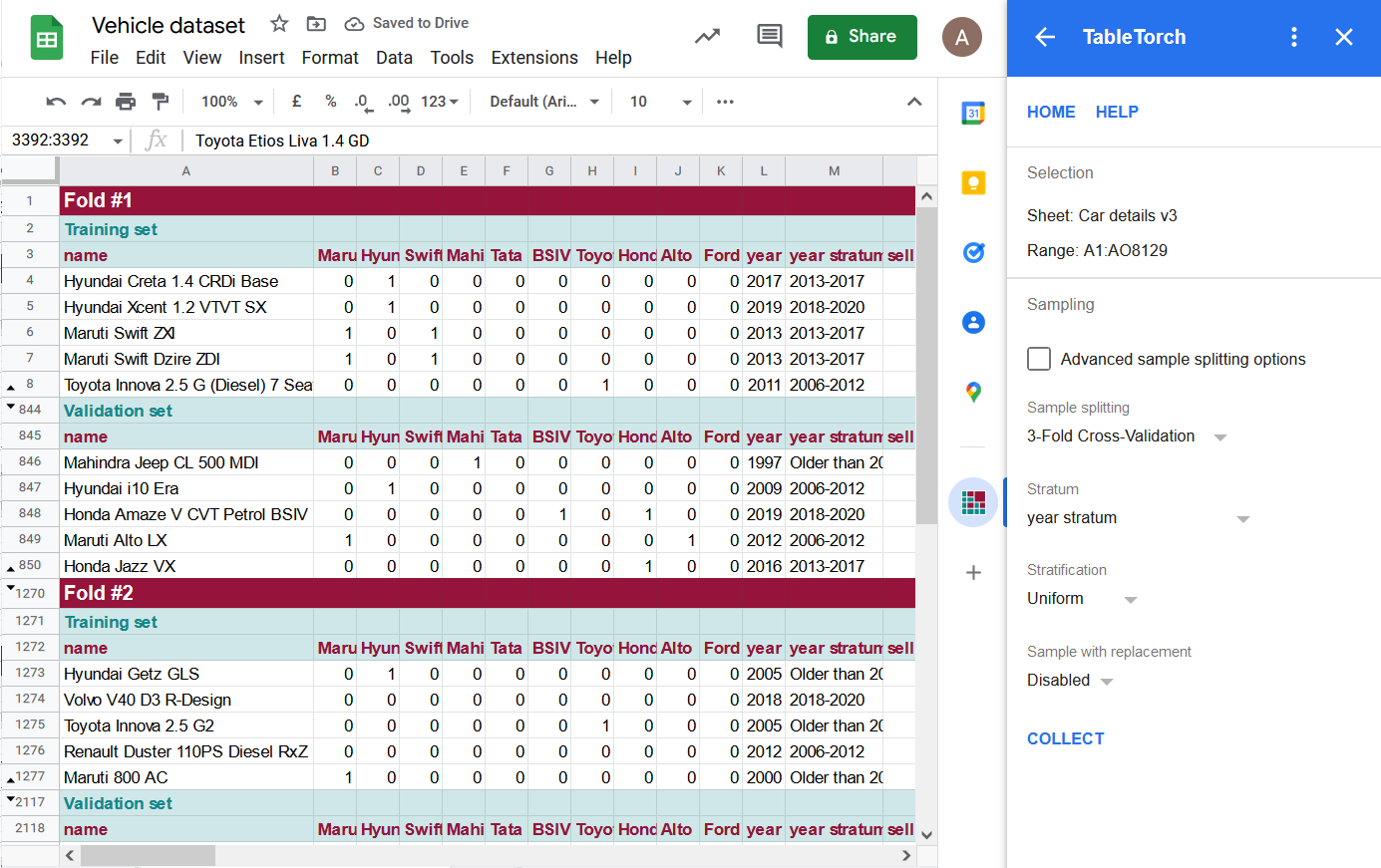Some of the rows are hidden on the picture above so that the header rows of the folds and their sets are seen.

TableTorch produced 3 folds, each of them contains a training set with two thirds of the data and a validation set containing a unique one third of original dataset.

Each stratum identified by the year stratum column is uniformly represented in a each of the training and validation sets, i.e. the number of rows should be the same. A slight deviation may occur if the number of rows cannot be divided by cross-validation’s k parameter (i.e. 3, 5, or 10) evenly.

## Sampling with replacement

Replacement allows performing stratified random sampling without worrying about the underrepresented strata.

Imagine a dataset of 200 rows where 40 rows belong to stratum A and 160 to stratum B. Stratified uniform random sampling should produce a dataset with identical number of rows belonging to each stratum. Hence with default settings, it can only produce a dataset consisting of 80 records, 40 of A and 40 of B. Any statistical analysis to be done on the produced sample will lose 120 or 75% of the rows belonging to stratum B which is significant signal loss and might impair soundness of the analysis.

Sampling with replacement is designed to alleviate this shortcoming. It does so by randomly selecting a row from the original set a predefined number of times. Thus, if replacement is used, stratified random uniform sampling can produce a dataset with 160 or more rows for both strata. However, some of those rows will be duplicates so these kind of sampling is only suitable for certain statistical analysis, e.g. for linear regressions.

If replacement is enabled, TableTorch uses the following heuristic to compute the number of rows to select:

• Let n be the number of rows in original dataset divided by the count of strata. E.g. for a dataset of 240 rows and 3 strata, n is 80.
• If original dataset has less than 1000 rows, it selects a multiple of n rows so as to increase chances of all of the original rows getting into the dataset. For example, if n is 80, TableTorch is likely to select at least 200 rows for each stratum depending on the number of columns and other circumstances.
• Otherwise, it selects n rows for each stratum. This may result into some of the rows being omitted from the resulting sample, however it is needed to reduce the probability of exceeding maximum execution time or inadvertently adding more cells than 5 million cells limit of Google Sheets.

## Formulas

TableTorch copies the data into resulting dataset by values, i.e. formulas are not copied. This is done to speed up the process and avoid exceeding quotas. It is deemed that the produced sheet is of a temporary nature and is useful for subsequent regressions or other data manipulation rather than formula experimentation.